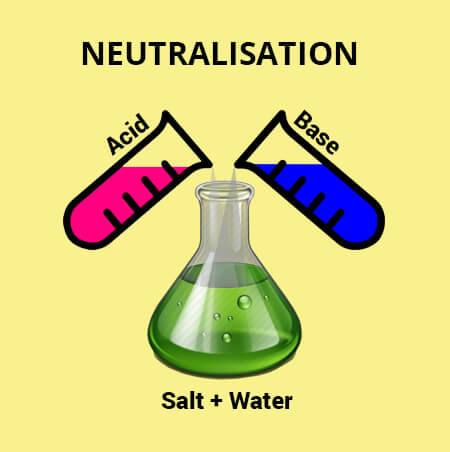# Neutralization Reaction Calculator

Find the equivalent base of acid or base using Neutralization Reaction Calculator, which is also known as acid base reaction calculator.

Formula Normality = Weight Volume * Equivalent Weight## Neutralization reaction Formula

The formula for neutralization reaction is:

Equivalent weight of acid or base = Weight of acid or base in gramsVolume of base or acid × Normality

Acid base neutralization calculator uses the above equation for the calculations of neutralization reaction.

## How acid-base reaction calculator works?

The neutralization reaction equation calculator simply takes the input from the user and produces very accurate results.

To use the neutralize calculator, follow the below steps.

• Enter the weight of acid or base in the given input box.
• Enter the volume of base or acid and normality as well.
• Hit the Calculate button. Use the Reset button to enter new values.

## What is a neutralization reaction?

Neutralization refers to a chemical reaction in which an acid and a base interact to form water and salt as products.The method is based on the fact that one gram equivalent of the acid completely neutralizes one gram equivalent of the base and vice versa.

For example:

HCL (acid) + NaOH (base) H2O (water) + NaCl (salt)

Neutralization equation calculator calculates the equivalent weight of acid/base in neutralization reactions like mentioned above.## How to find equivalent weight in neutralization reaction?

A reaction between an acid and a base that yields a salt and water is termed as neutralization. Here's how you can calculate the equivalent weight of acid or base without using acid neutralization calculator.

Example:
Calculate the equivalent weight of acid or base if the weight of acid or base is 25 grams, volume of base or acid is 30, and normality is 15.

Solution:

Step 1: Identify and write down the given values.

Weight of acid or base in grams = 25
Volume of base or acid = 30
Normality = 15

Step 2: Apply formula and place the values.

Equivalent weight of acid or base = Weight of acid or base in gramsVolume of base or acid × Normality

Equivalent weight of acid or base = 2530 × 15

Equivalent weight of acid or base = 12.5

## Neutralization reaction examples

Here are a few examples of neutralization reactions. Use the chemistry neutralization calculator to find the equivalent weight of acid/base for the given examples.

HCl + NaOH → NaCl + HOH
H2SO4 + 2 NH4OH → (NH4)2SO4 + 2 HOH
2 NaOH + H2CO3 → N2CO3 + 2 NaOH
Ca (OH)2 + H2CO3 → CaCO3 + 2 HOH

1. Neutralization. Chemistry LibreTexts.
2. Key J, Ball D. Neutralization Reactions. Ecampusontario.pressbooks.pub